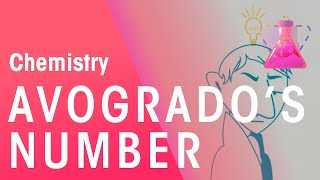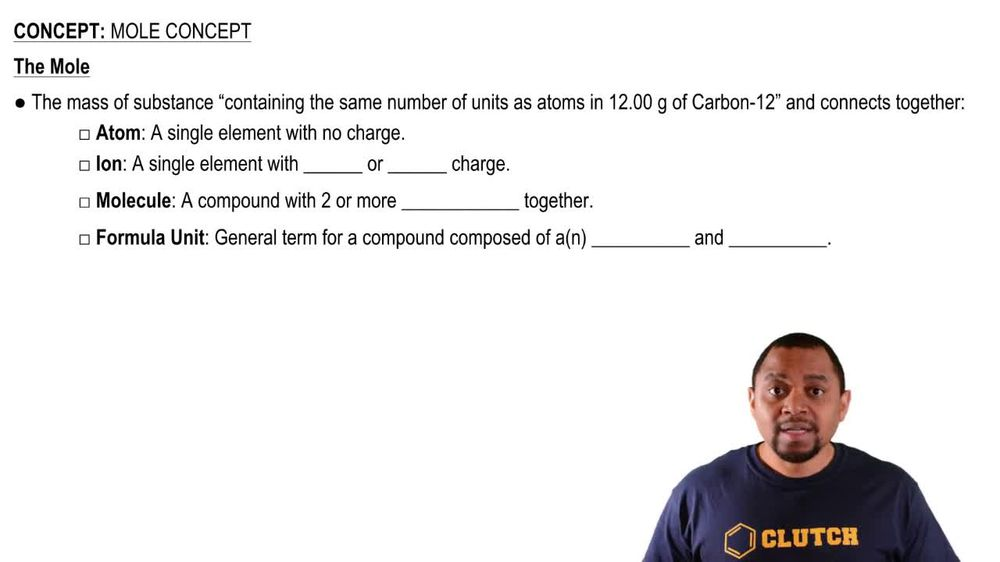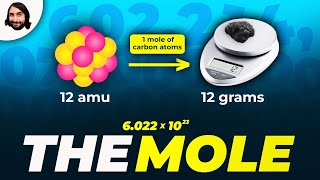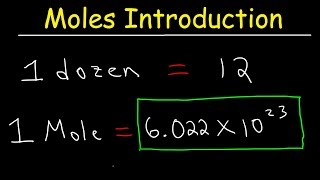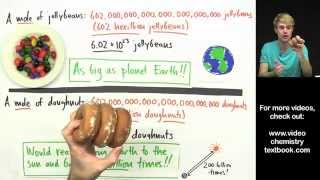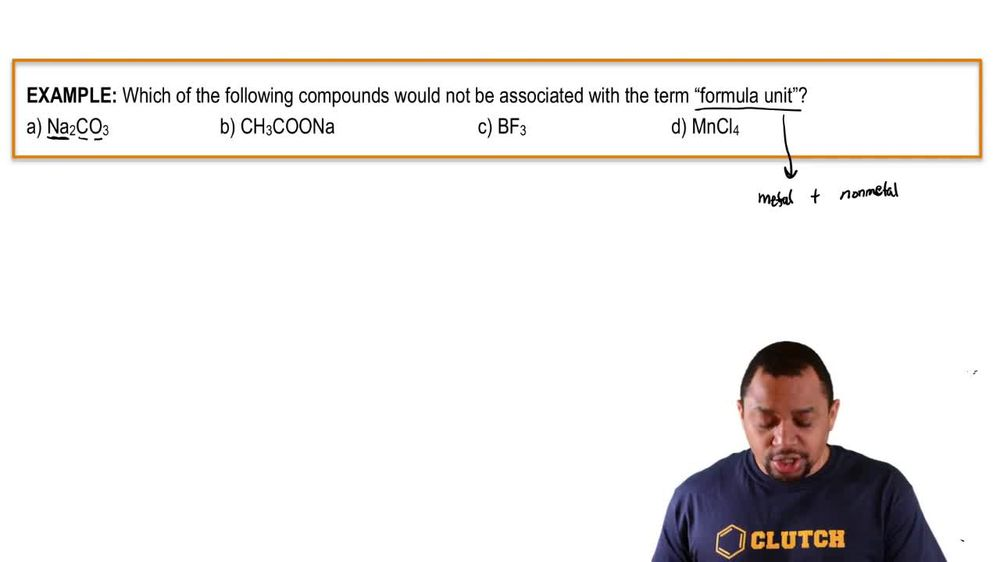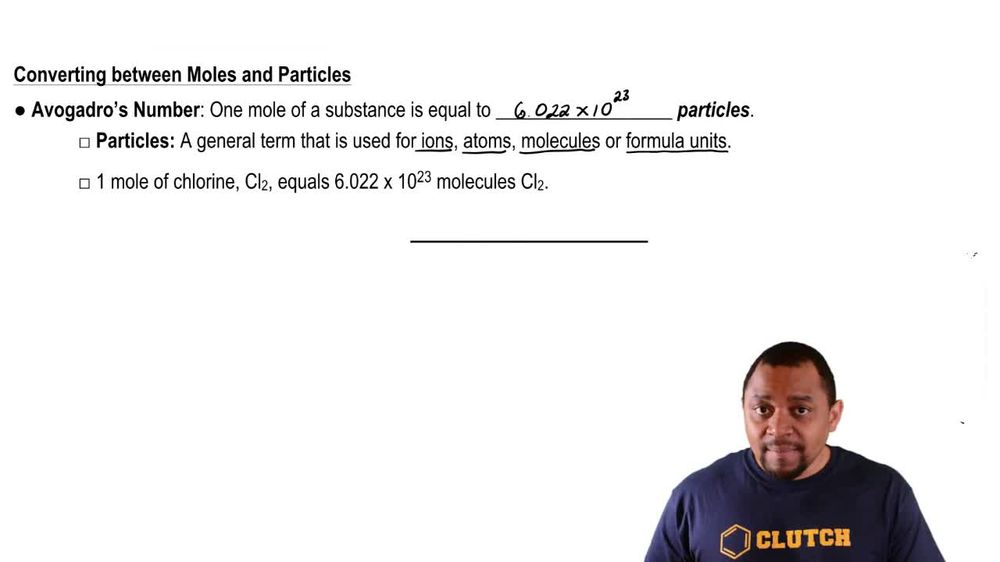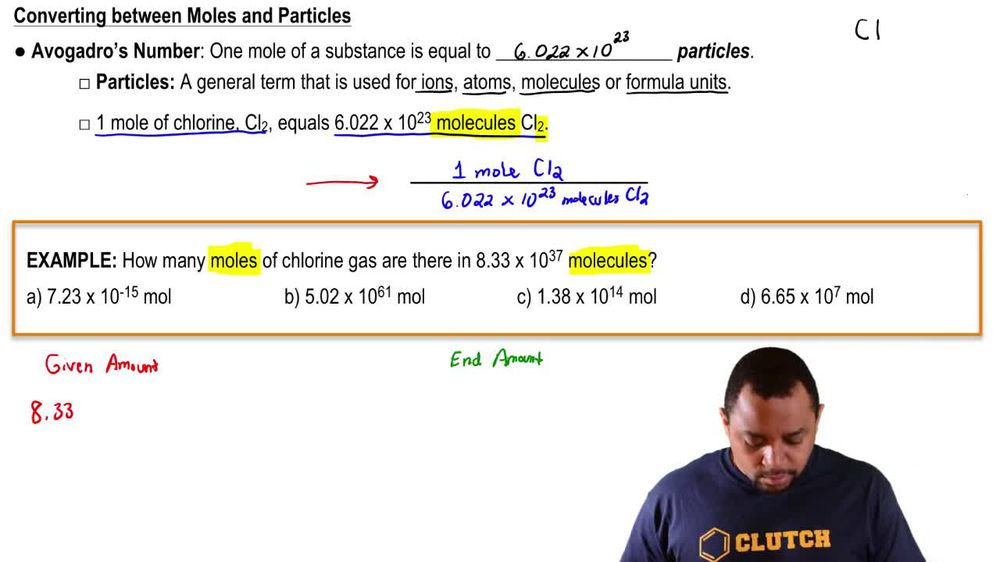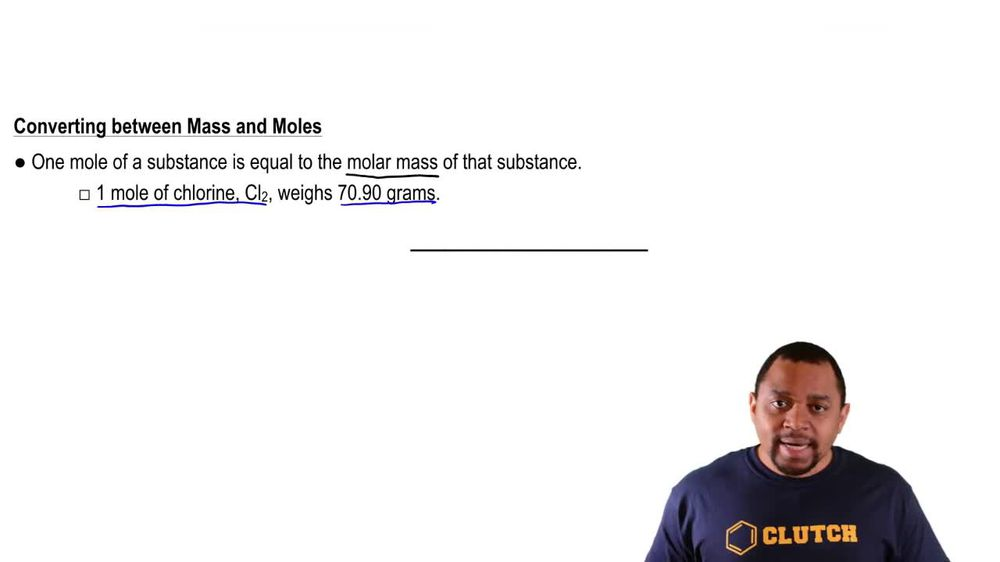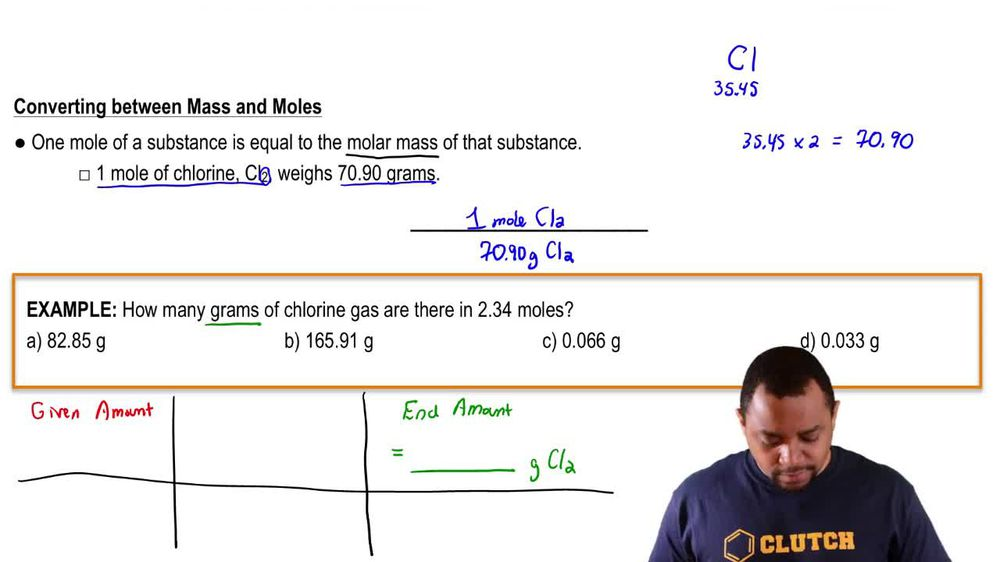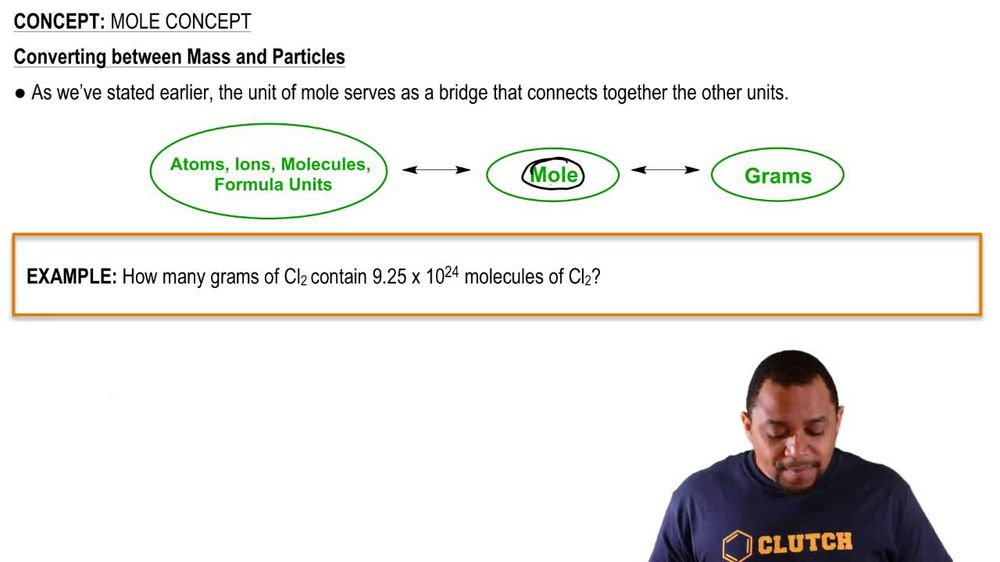Start typing, then use the up and down arrows to select an option from the list.
1. 2. Atoms & Elements2. Mole Concept
Problem

# What is the amount, in moles, of each elemental sample? a. 11.8 g Ar

Relevant Solution6m
Play a video:
Hi everyone for this problem. We want to know how many kilograms of carbon Does 50 kg of this compound contain. So let's start off by writing down what's given. We know that we have 50 kg of this compound and we want to know specifically how many kilograms of carbon is in this compound. So we can use stoke eom a tree and dimensional analysis to be able to solve this problem. The first thing that we're going to want to do is get into a unit of grams. How many grams of our compound do we have? Because we're going to most likely be using molar mass here and molar mass is in units of grams per mole. So let's go ahead and convert this from kilograms of our compound, two g and one kg of our compound, we have grants of our compound. Okay? So if we pay attention to our units here are kilograms cancel. And now we're in units of grams. Now we want to go from grams of our compound to moles of our compound. To be able to use a multiple ratio between our entire compound and carbon specifically. So let's do that by finding the molar mass of our entire compound. So our molar mass of our compound is equal to, We have three carbons And each one is 12.01 g. So we have a total of 36.03 g of carbon. And we have two florins Which each weigh 19 g. So we have a total of 38 g of flooring. And we have six quarantines, which each way 35. grams. This gives us a total of 212.7 g. And this is all on our periodic table. So once we add all of this up we get a molar mass of 286. grams per mole of our compound. So we can plug that in now into our table and we can say that. and one mole of our compound, we have 0.73 g per. We have, yeah, we have 200 and 86.73 g of our compound. Okay. And so now our grams of our compound cancel. And now we're in moles of our compound and we want to go from moles of our compound to moles of carbon. So let's use our multiple ratio Using the formula that's given. So we can say that and one more of our compound. How many moles of carbon do we have? We have three. There's three malls of carbon. So now we are in now we know what our moles of carbon is because our molds of our compound cancel. And now we need to go from moles of carbon to grams of carbon. And we can do that using our Mueller mass of carbon. So in one mole of carbon we have 0.1 grams of carbon. Our modes of carbon cancel. Now the question asked us, how many kilograms of carbon does this sample contain? So the last thing that we need to do is go from grams of carbon to kilograms. So in one kg of carbon we have 1000 grams of carbon. Okay, so let's make sure we cross that unit out. So now we have our final unit of kilograms of carbon. And all we have to do now is the math. And once we do this math, we're left with a final answer of 6. kilograms of carbon. This is our final answer. This is the Amount of carbon and kg and 50 kg of our sample. Okay, that's the end of this problem. I hope this was helpful.